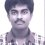# Help: Equilibrium 1

$40 mL$, $0.1 M$ ammonia solution is mixed with $20 mL$ $0.1 M HCl$ , what is the $pH$ of mixture ?($pK_{a}$ of $NH_{3}$ solution =$4.74$)

Now, what I did was as follows:-

First of all I calculated the $[OH^{-}]$ obtained from the ammonia solution. Which i did as follows $NH_{ 3 }\quad \quad \quad \quad +\quad \quad \quad \quad H_{ 2 }O\quad \quad \quad \quad \leftrightharpoons \quad \quad \quad \quad NH_{ 4 }^{ + }\quad \quad \quad \quad +\quad \quad \quad \quad { OH }^{ - }\\ \quad c\quad \quad \quad \quad \quad \quad \quad \quad \quad \quad \quad \quad \quad \quad \quad \quad \quad \quad \quad \quad \quad \quad \quad \quad \quad 0\quad \quad \quad \quad \quad \quad \quad \quad \quad \quad \quad \quad 0\\ c-c\alpha \quad \quad \quad \quad \quad \quad \quad \quad \quad \quad \quad \quad \quad \quad \quad \quad \quad \quad \quad \quad \quad \quad \quad c\alpha \quad \quad \quad \quad \quad \quad \quad \quad \quad \quad \quad c\alpha$

From above we can conclude that $\left[ { OH }^{ - } \right] =\sqrt { { K }_{ b }c }$ (We get ${ K }_{ b }$ from the relation ${ K }_{ b }+{ K }_{ c }=14$)

The explanation is getting bigger so i will cut to the chase.

After obtaining the $\left[ { OH }^{ - } \right]$, I found the moles of ${ OH }^{ - }$ and subtracted it from the moles of ${ H }^{ + }$ and the and found the conc. of ${ H }^{ + }$, then the -ve logarithm. But the book gives a different answer from what i got. So if anything that i did wrong or left somewhere could you point it out4 years, 7 months ago

This discussion board is a place to discuss our Daily Challenges and the math and science related to those challenges. Explanations are more than just a solution — they should explain the steps and thinking strategies that you used to obtain the solution. Comments should further the discussion of math and science.

When posting on Brilliant:

• Use the emojis to react to an explanation, whether you're congratulating a job well done , or just really confused .
• Ask specific questions about the challenge or the steps in somebody's explanation. Well-posed questions can add a lot to the discussion, but posting "I don't understand!" doesn't help anyone.
• Try to contribute something new to the discussion, whether it is an extension, generalization or other idea related to the challenge.

MarkdownAppears as
*italics* or _italics_ italics
**bold** or __bold__ bold
- bulleted- list
• bulleted
• list
1. numbered2. list
1. numbered
2. list
Note: you must add a full line of space before and after lists for them to show up correctly
paragraph 1paragraph 2

paragraph 1

paragraph 2

[example link](https://brilliant.org)example link
> This is a quote
This is a quote
    # I indented these lines
# 4 spaces, and now they show
# up as a code block.

print "hello world"
# I indented these lines
# 4 spaces, and now they show
# up as a code block.

print "hello world"
MathAppears as
Remember to wrap math in $$ ... $$ or $ ... $ to ensure proper formatting.
2 \times 3 $2 \times 3$
2^{34} $2^{34}$
a_{i-1} $a_{i-1}$
\frac{2}{3} $\frac{2}{3}$
\sqrt{2} $\sqrt{2}$
\sum_{i=1}^3 $\sum_{i=1}^3$
\sin \theta $\sin \theta$
\boxed{123} $\boxed{123}$

Sort by:

Your equilibrium condition is not considering HCl. The equilibrium shifts much more to the right to produce hydroxide ions in presence of the acidic HCl. This solution acts as a buffer solution.

- 4 years, 7 months ago

@Rishabh Cool. If you can also look into this one please.

- 4 years, 7 months ago